# How to negate grid like blocks without unions?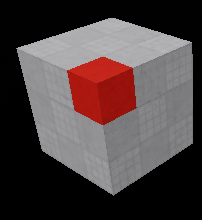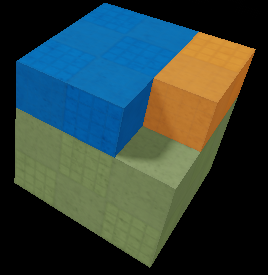How would I negate block like parts, without using unions but instead splitting it into other parts as shown above?

1 Like

EDIT: Okay, I posted the second part that actually solves what you were asking forWhat a fun problem! Hope this is of some help.

EDIT: I swapped ∪ for ∩ >.<’ it’s fixed now tho

EDIT: Here's the full script, with added comments and type annotations:
``````function corner1(part)
return part.Position - part.Size / 2
end

function corner2(part)
return part.Position + part.Size / 2
end

function minMax(v1, v2)
return math.min(v1, v2), math.max(v1, v2)
end

function avg(v1, v2)
return (v1 + v2) / 2
end

--[[
Given two number ranges (p11, p12) and (p21, p22), return the intersection of those ranges.
]]
function numbersIntersection(p11: number, p12: number, p21: number, p22: number): {number}
--Ensure segments (p11, p12) and (p21, p22) go from small to large
--Ensure p11 comes before p12
p11, p12 = minMax(p11, p12)
--Ensure p21 comes before p22
p21, p22 = minMax(p21, p22)

--Ensure (p11, p12) starts before (p21, p22)
if p11 > p21 then
--... by swapping if that's not the case
p11, p12, p21, p22 = p21, p22, p11, p12
end

if p12 < p21 then
return {} --No overlap
elseif p12 == p21 then
return {p12} --Overlaps in a point
elseif p12 > p21 then
if p12 < p22 then
return {p21, p12} --Some overlapping segment
else
return {p21, p22} --Entirity of (p21, p22) is contained in (p11, p12)
end
else
error()
end
end

--[[
Given two number ranges (p11, p12) and (p21, p22), return the second range removed from the first.
]]
function numbersDifference(p11: number, p12: number, p21: number, p22: number): {number}
--Ensure segments (p11, p12) and (p21, p22) go from small to large
--Ensure p11 comes before p12
p11, p12 = minMax(p11, p12)
--Ensure p21 comes before p22
p21, p22 = minMax(p21, p22)

--Nothing or everything gets deleted
local intersection = numbersIntersection(p11, p12, p21, p22)
if #intersection == 0 then --No overlap
return {p11, p12} --Just return first segment
elseif intersection == p11 and intersection == p12 then --Overlap is entire first segment
return {} --Nothing left
end

--Something but not everything gets deleted
if p21 <= p11 then -- (p21, p22) starts before (p11, p12) and ends inside
--Only 1 case because if p22 were >= p12, everything would get deleted and that's checked previously
return {p11, p22, p12}, 1 --First part gets deleted, second part stays
elseif p22 >= p12 then -- (p21, p22) starts inside (p11, p12) and ends outside then
--Only 1 case because if p21 were <= p11, everything would get deleted and that's checked previously
return {p11, p21, p12}, 2  --Second part gets deleted, first part stays then
else --Second segment is inside first segment, so cut out middle part
return {p11, p21, p22, p12}, 2 --Middle gets deleted, other parts stay
end
end

--[[
Return a non-rotated Part with Size and Position defined by two corners.
Optionally set the Size and Position of an existing Part, or use a new Part.
]]
function partFromCorners(corner1: Vector3, corner2: Vector3, existingPart: Part?): Part
local part = existingPart or Instance.new("Part")

local diff = corner2 - corner1
local mid = avg(corner1, corner2)

part.Size = Vector3.new(diff.X, diff.Y, diff.Z)
part.Position = Vector3.new(mid.X, mid.Y, mid.Z)

return part
end

--[[
Given a non-rotated Part, an Axis and a list of points along that axis (as numbers),
return the slices of the Part on the plane perpendicular to the axis and intersecting the points
along the axis, as a list of Parts.
]]
function slicePart(part: Part, axis: Enum.Axis, points: {number})
local slices = {}

local cutAxis = Vector3.FromAxis(axis)
local cutPlane = Vector3.new(1, 1, 1) - cutAxis

local sCorner1 = corner1(part)
local sCorner2 = sCorner1 * cutAxis + corner2(part) * cutPlane --Gets zero'd on the cut axis (so it corner2 on cut axis = corner 1 on cut axis)

for i = 1, #points do
sCorner2 += points[i] * cutAxis - sCorner1 * cutAxis --Slide corner2 to point[i] on the cut axis
table.insert(slices, partFromCorners(sCorner1, sCorner2, part:Clone()))
sCorner1 += (sCorner2 - sCorner1) * cutAxis --Slide corner1 to corner2 on the cut axis
end

sCorner2 = corner2(part)
table.insert(slices, partFromCorners(sCorner1, sCorner2, part:Clone()))

return slices
end

--[[
Given two volumes (as Parts), return the intersection of those volumes (as a Part).
Input parts must have no rotation.
]]
function volumesIntersection(block1: Part, block2: Part): Part?
local b1c1, b1c2 = corner1(block1), corner2(block1)
local b2c1, b2c2 = corner1(block2), corner2(block2)

local xDifference = numbersDifference(b1c1.X, b1c2.X, b2c1.X, b2c2.X)
local yDifference = numbersDifference(b1c1.Y, b1c2.Y, b2c1.Y, b2c2.Y)
local zDifference = numbersDifference(b1c1.Z, b1c2.Z, b2c1.Z, b2c2.Z)

--If any axis is non-intersecting, the intersection is empty
if #xDifference ~= 2 and #yDifference ~= 2 and #zDifference ~= 2 then return nil end

--If all axes are intersecting, return the 3D intersection
local corner1 = Vector3.new(xDifference, yDifference, zDifference)
local corner2 = Vector3.new(xDifference, yDifference, zDifference)

local difference = block1:Clone()
partFromCorners(corner1, corner2, difference)

return difference
end

--[[
Given two volumes (as Parts), return the second volume removed from the first volume (as a list of Parts).
Input parts must have no rotation.
]]
function volumesDifference(block1: Part, block2: Part): {Part}
local parts = {}

-- Compute the differences on each axis *before* slicing on the axes,
--	because we might exit early if one or more axes are non-overlapping
local axisDifferences = {}
for _, axis in pairs(Enum.Axis:GetEnumItems()) do
local A = axis.Name
local points, toDelete = numbersDifference(corner1(block1)[A], corner2(block1)[A], corner1(block2)[A], corner2(block2)[A])

axisDifferences[axis] = {points = points, toDelete = toDelete}
if #points == 2 then --Or equivalently if toDelte == nil, i.e. block2 and block1 do not intersect.
return { block1:Clone() }
end
end

-- Slice along axes after verifying that none are non-interesecting
for _, axis in pairs(Enum.Axis:GetEnumItems()) do
local A = axis.Name
local differences = axisDifferences[axis]
local points: {number}, toDelete = differences.points, differences.toDelete
print(points)
--Slices are to be made between each point, but slicePart expects a list of points to slice AT, not two points to slice BETWEEN.
--Fix this by removing first and last points.
table.remove(points, #points)
table.remove(points, 1)

--Skip if (block1 - block2) == block1, i.e. block2 is completely outside block 1
if #points == 0 then continue end

--Slice block1 according to where it intersects block2
local slices = slicePart(block1, axis, points)

--Return all the slices except the ones that are inside block2
for i = 1, #slices do
if i == toDelete then continue end
table.insert(parts, slices[i])
end
end

return parts
end
``````

# Part 1

## Problem description

First of all, I’m assuming the blocks will always be axis-aligned (i.e. not rotated).

What you want to find is `A - B`, where `A` is the grey block and `B` is the red one. As you’ve shown it, `B` fits nicely inside `A`, but that might not always be the case. We might as well solve the problem in a more general case where only some of `B` overlaps `A`, or even where they’re not even touching. In that case, what you want to do is remove from `A` the volume that is shared between `A` and `B`, which can be written as `A ∩ B` (a symbol from set theory meaning “intersection”). So the first step is to find `A ∩ B`.

## Solution in 1D

It’s helpful to solve it first in 1D and then extend the solution to 3D, because 1D is much simpler and easier to think about. In 1D there are a few cases that must be handled differently.

I've illustrated all the cases below:

Case 1 (no overlap)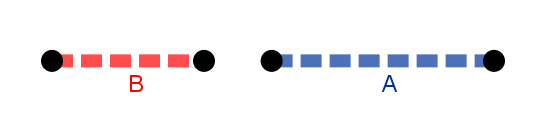Case 2 (overlap in a point)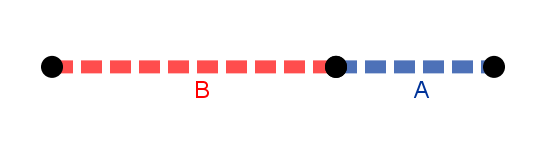Case 3 (overlap in a segment)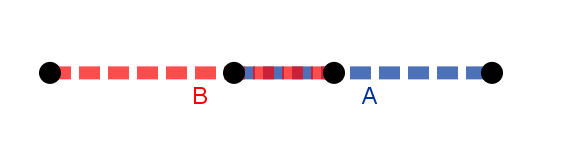Case 4 (A completely inside B)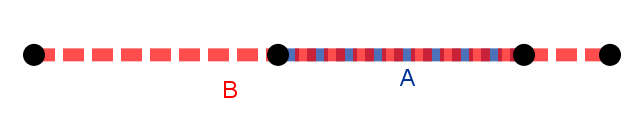You might wonder why there are not cases where A is to the left of B. That’s because `∩` is completely symmetric, i.e. `A ∩ B = B ∩ A`. For example this case…

is the same as case 1 except the colors and labels are switched, but because of that property of `∩` it’s the same as case 1.

Since we’re working in 1D the points are just numbers on the number line, so let’s create a function called `numbersIntersection` that finds `A ∩ B` where `A=(p11, p12)` and `B=(p21, p22)`:

``````function numbersIntersection(p11, p12, p21, p22)
-- ... something something
-- return {point1 of A ∩ B, point 2 of A ∩ B}
end
``````

The function will return a table containing 0, 1 or 2 points (which are numbers because 1D) that make up `A ∩ B`.

It's helpful to swap the parameters around to make sure they're always in the order described:
``````function minMax(v1, v2)
return math.min(v1, v2), math.max(v1, v2)
end

function numbersIntersection(p11, p12, p21, p22)
--Ensure segments (p11, p12) and (p21, p22) go from small to large
--Ensure p11 comes before p12
p11, p12 = minMax(p11, p12)
--Ensure p21 comes before p22
p21, p22 = minMax(p21, p22)

--Ensure (p11, p12) starts before (p21, p22)
if p11 > p21 then
--... by swapping if that's not the case
p11, p12, p21, p22 = p21, p22, p11, p12
end

-- ... return something
end
``````

-

And now it's just a matter of returning the right segment in each of the 4 cases:
``````function numbersIntersection(p11, p12, p21, p22)
--Ensure segments (p11, p12) and (p21, p22) go from small to large
--Ensure p11 comes before p12
p11, p12 = minMax(p11, p12)
--Ensure p21 comes before p22
p21, p22 = minMax(p21, p22)

--Ensure (p11, p12) starts before (p21, p22)
if p11 > p21 then
--... by swapping if that's not the case
p11, p12, p21, p22 = p21, p22, p11, p12
end

if p12 < p21 then --End of segment 1 comes before start of segment 2
return {} --No overlap
elseif p12 == p21 then --End of segment 1 is same as start of segment 2
return {p12} --Overlaps in a point
elseif p12 > p21 then --End of segment 1 inside segment 2
if p12 < p22 then --Start of segment 1 before start of segment 2
return {p21, p12} --Some overlapping segment
else --Start of segment 1 inside segment 2
return {p21, p22} --Entirity of (p21, p22) is contained in (p11, p12)
end
end
end
``````

## Solution in 2D

The intersection of two 1D segments was (at most) a 1D segment made up of two numbers.

Likewise, the intersection of two 2D rectangles is (at most) a 2D rectangle made up of 2 1D segments at right angles (because we know they’re not rotated), or 4 numbers (start and end on X axis, start and end on Y axis). Once again because we know the rectangles are axis aligned we don’t actually need all 4 points, we can figure out the rest if we just have 2 opposing corners.

The 2D solution illustrated:

The intersection is the rectangle made up of the two segments (projected onto the X and Y axes in this illustration). We can find those segments as the intersections of the vertical and horizontal parts of the rectangles.

## Solution in 3D

The intersection of two 3D cuboids is at most a 3D cuboid made up of 3 1D segments at right angles, or 6 numbers. Once again we only need the two opposing corners. We can find those segments as the intersections of the vertical, horizontal and depth-wise parts of the cuboids.

In code that looks like this (full script):
``````
function corner1(part)
return part.Position - part.Size / 2
end

function corner2(part)
return part.Position + part.Size / 2
end

function minMax(v1, v2)
return math.min(v1, v2), math.max(v1, v2)
end

function avg(v1, v2)
return (v1 + v2) / 2
end

function numbersIntersection(p11, p12, p21, p22)
--Ensure segments (p11, p12) and (p21, p22) go from small to large
--Ensure p11 comes before p12
p11, p12 = minMax(p11, p12)
--Ensure p21 comes before p22
p21, p22 = minMax(p21, p22)

--Ensure (p11, p12) starts before (p21, p22)
if p11 > p21 then
--... by swapping if that's not the case
p11, p12, p21, p22 = p21, p22, p11, p12
end

if p12 < p21 then
return {} --No overlap
elseif p12 == p21 then
return {p12} --Overlaps in a point
elseif p12 > p21 then
if p12 < p22 then
return {p21, p12} --Some overlapping segment
else
return {p21, p22} --Entirity of (p21, p22) is contained in (p11, p12)
end
end
end

function partFromCorners(corner1, corner2, part)
local part = part or Instance.new("Part")

local diff = corner2 - corner1
local mid = avg(corner1, corner2)

part.Size = Vector3.new(diff.X, diff.Y, diff.Z)
part.Position = Vector3.new(mid.X, mid.Y, mid.Z)

return part
end

function volumesIntersection(block1: Part, block2: Part)
local b1c1, b1c2 = corner1(block1), corner2(block1)
local b2c1, b2c2 = corner1(block2), corner2(block2)

local xIntersection = numbersIntersection(b1c1.X, b1c2.X, b2c1.X, b2c2.X)
local yIntersection = numbersIntersection(b1c1.Y, b1c2.Y, b2c1.Y, b2c2.Y)
local zIntersection = numbersIntersection(b1c1.Z, b1c2.Z, b2c1.Z, b2c2.Z)

if #xIntersection == 2 and #yIntersection == 2 and #zIntersection == 2  then
local corner1 = Vector3.new(xIntersection, yIntersection, zIntersection)
local corner2 = Vector3.new(xIntersection, yIntersection, zIntersection)

local intersection= block1:Clone()
partFromCorners(corner1, corner2, intersection)

return intersection
end
end
``````

## Part 1 results

Testing it I get this: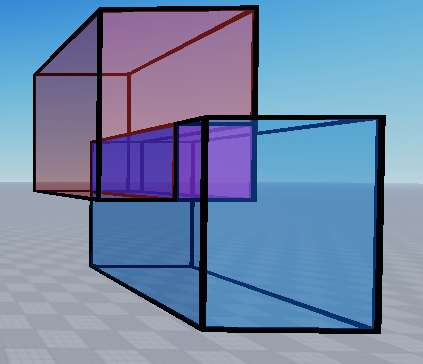... with this code:
``````local a, b = game.Workspace.A, game.Workspace.B
local u = volumesIntersection(a, b)
u.Name = "A∩B"
u.Color = Color3.fromRGB(255, 0, 255)
u.Parent = game.Workspace
``````
4 Likes

# Part 2

## Problem description

Now that we can calculate `A ∩ B`, it’s not so difficult to calculate `A - B`.

## Solution in 1D

In the case where there’s no overlap, the result of the operation is to just leave A be, because B doesn’t “take a bite” out of it.

That leaves these 3 cases:

Cases 1 and 2: A is cut into 2 segments, where either the first or last one is deleted.

Case 3: A gets cut into 3 segments where the middle one is deleted.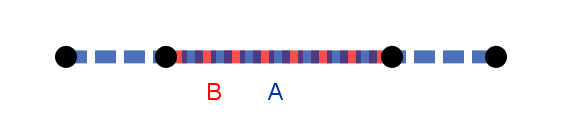Case 4: A is entirely eaten by B so there are 0 segments left: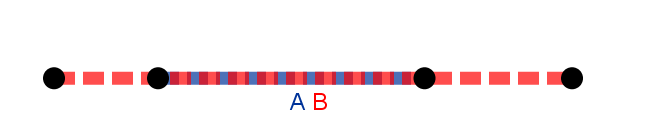## Solution in 2D

Let’s look at case 1 in 2D:

If we were to think of how we’d apply the 1D solution to figure out the 4 - 1 = 3 rectangles to create in 2D, we’d first cut `A` into 2 rectangles along one axis, leave one of them be and then cut the other into 2 rectangles on the other axis. This gives us 3 different parts of which 1 must be deleted.

## Solution in 3D

To create the parts we need in 3D we use the same logic: cut first on one axis, then another axis, and then the final axis: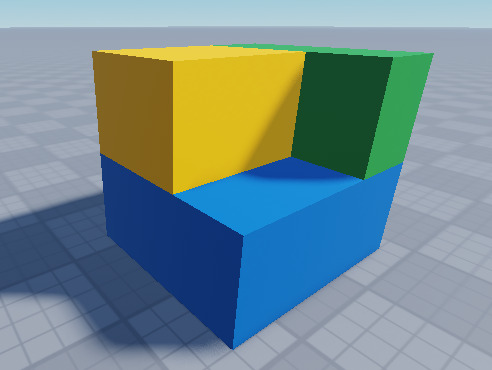In this image I first cut the part on the Y axis, then one of the horizontal axes and then the other. So first I turned the blue box that was the entire part into 2 boxes, coloring the top one green. Then I cut the green in two, coloring one of the parts yellow. Then I cut the yellow box in 2 and deleted one of the yellow parts.

Examples

A similar logic applies for case 3 where the middle must be removed. Notice that the 3 cases can be mixed and matched between the 3 axes, e.g.:

Cutting a hole in a part: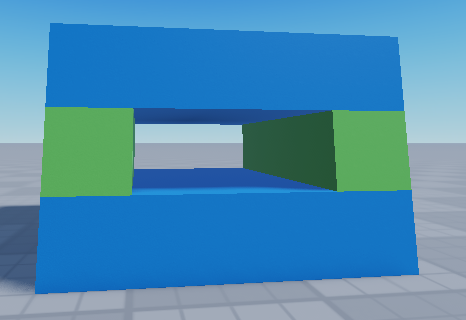3 blue segments, middle gets deleted. 3 green segments, middle gets deleted. 0 yellow segments because B completely ate A on that axis.

Cutting a notch into a part:

B only ate one end of A on that axis:## 1D code

For the same reasons as in part 1, we fix the order of the parameters so they’re always right. Except we don’t swap which segment is which, because `A - B ~= B - A`.

Swapping
``````function numbersSub(p11, p12, p21, p22)
--Ensure segments (p11, p12) and (p21, p22) go from small to large
--Ensure p11 comes before p12
p11, p12 = minMax(p11, p12)
--Ensure p21 comes before p22
p21, p22 = minMax(p21, p22)
...
end
``````
Then we handle the situations where everything or nothing gets eaten:
``````function numbersSub(p11, p12, p21, p22)
--Ensure segments (p11, p12) and (p21, p22) go from small to large
--Ensure p11 comes before p12
p11, p12 = minMax(p11, p12)
--Ensure p21 comes before p22
p21, p22 = minMax(p21, p22)

--Nothing or everything gets deleted
local intersection= numbersIntersection(p11, p12, p21, p22)
if #intersection== 0 then --No overlap
return {p11, p12} --Just return first segment
elseif intersection == p11 and intersection == p12 then --Overlap is entire first segment
return {} --Nothing left
end
...
``````
and finally we handle the 3 cases:
``````function numbersSub(p11, p12, p21, p22)
--Ensure segments (p11, p12) and (p21, p22) go from small to large
--Ensure p11 comes before p12
p11, p12 = minMax(p11, p12)
--Ensure p21 comes before p22
p21, p22 = minMax(p21, p22)

--Nothing or everything gets deleted
local intersection= numbersIntersection(p11, p12, p21, p22)
if #intersection== 0 then --No overlap
return {p11, p12} --Just return first segment
elseif intersection == p11 and intersection == p12 then --Overlap is entire first segment
return {} --Nothing left
end

--Something but not everything gets deleted
if p21 <= p11 then -- (p21, p22) starts before (p11, p12) and ends inside
--Only 1 case because if p22 were >= p12, everything would get deleted and that's checked previously
return {p11, p22, p12}, 1 --First part gets deleted, second part stays
elseif p22 >= p12 then -- (p21, p22) starts inside (p11, p12) and ends outside then
--Only 1 case because if p21 were <= p11, everything would get deleted and that's checked previously
return {p11, p21, p12}, 2  --Second part gets deleted, first part stays then
else --Second segment is inside first segment, so cut out middle part
return {p11, p21, p22, p12}, 2 --Middle gets deleted, other parts stay
end
end
``````

## 3D code

Now we need a way of cutting a block into smaller blocks on any axis. Here's a function that takes a part, an axis to slice along, and points along that axis to slice at:
``````function slicePart(part: Part, axis: Enum.Axis, points)
local slices = {}

local cutAxis = Vector3.FromAxis(axis)
local cutPlane = Vector3.new(1, 1, 1) - cutAxis

local sCorner1 = corner1(part)
local sCorner2 = sCorner1 * cutAxis + corner2(part) * cutPlane --Gets zero'd on the cut axis (so it corner2 on cut axis = corner 1 on cut axis)

for i = 1, #points do
sCorner2 += points[i] * cutAxis - sCorner1 * cutAxis --Slide corner2 to point[i] on the cut axis
table.insert(slices, partFromCorners(sCorner1, sCorner2, part:Clone()))
sCorner1 += (sCorner2 - sCorner1) * cutAxis --Slide corner1 to corner2 on the cut axis
end

sCorner2 = corner2(part)
table.insert(slices, partFromCorners(sCorner1, sCorner2, part:Clone()))

return slices
end
``````

It keeps track of two corners to create the slices at. They both start off at one end of the part, but still opposite each other (i.e. as opposite corners on one face of the part). The slice is then “walked along” the axis, first moving `sCorner2` to the next point, then creating the slice, then moving `sCorner1` up to `sCorner2` along the cut axis, kind of like a worm or something.

Aaaaand the logic for slicing `A` and deleting the part inside `B` looks like this:
``````function volumesSub(block1: Part, block2: Part)
local b1c1, b1c2 = corner1(block1), corner2(block1)
local b2c1, b2c2 = corner1(block2), corner2(block2)

local parts = {}

for _, axis in pairs(Enum.Axis:GetEnumItems()) do
local A = axis.Name
local points, toDelete = numbersSub(b1c1[A], b1c2[A], b2c1[A], b2c2[A])

--Slices are to be made between each point, but slicePart expects a list of points to slice AT, not two points to slice BETWEEN.
--Fix this by removing first and last points.
table.remove(points, #points)
table.remove(points, 1)

--Skip if (block1 - block2) == block1, i.e. block2 is completely outside block 1
if #points == 0 then continue end

--Slice block1 according to where it intersects block2
local slices = slicePart(block1, axis, points)

--Return all the slices except the ones that are inside block2
for i = 1, #slices do
if i == toDelete then continue end
table.insert(parts, slices[i])
end
end

return parts
end
``````

## Part 2 results

Testing it like this:
``````local a, b, c, d = game.Workspace.A, game.Workspace.B, game.Workspace.C, game.Workspace.D
local u = volumesIntersection(a, b)
u.Name = "A∩B"
u.Color = Color3.fromRGB(255, 0, 255)
u.Parent = game.Workspace

local s = volumesSub(c, d)
for _, part in pairs(s) do
part.Parent = game.Workspace
part.Transparency = 0
end
``````
I get these results: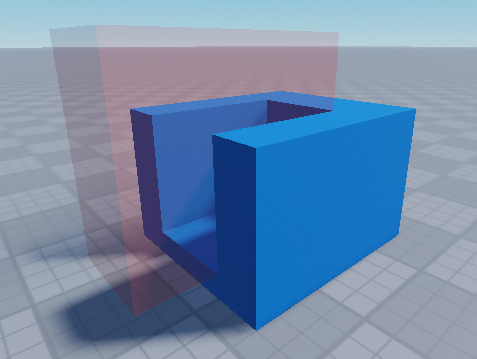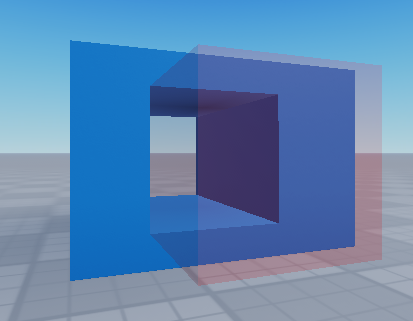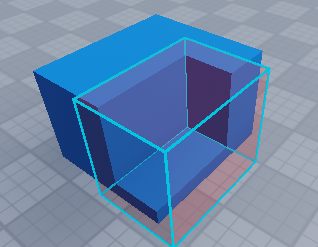3 Likes

How would I make it so the s parts wont collide with eachother, without re cutting them?. would be highly appreciatedAh, looks like a bugI’ll take a look when I have time

1 Like

Yeah, there was a bug where it kept reusing the original block to slice each axis, when it should have been using the results of slicing from the previous axis.

Here's a fixed version:
``````function corner1(part)
return part.Position - part.Size / 2
end

function corner2(part)
return part.Position + part.Size / 2
end

function minMax(v1, v2)
return math.min(v1, v2), math.max(v1, v2)
end

function avg(v1, v2)
return (v1 + v2) / 2
end

--[[
Given two number ranges (p11, p12) and (p21, p22), return the intersection of those ranges.
]]
function numbersIntersection(p11: number, p12: number, p21: number, p22: number): {number}
--Ensure segments (p11, p12) and (p21, p22) go from small to large
--Ensure p11 comes before p12
p11, p12 = minMax(p11, p12)
--Ensure p21 comes before p22
p21, p22 = minMax(p21, p22)

--Ensure (p11, p12) starts before (p21, p22)
if p11 > p21 then
--... by swapping if that's not the case
p11, p12, p21, p22 = p21, p22, p11, p12
end

if p12 < p21 then
return {} --No overlap
elseif p12 == p21 then
return {p12} --Overlaps in a point
elseif p12 > p21 then
if p12 < p22 then
return {p21, p12} --Some overlapping segment
else
return {p21, p22} --Entirity of (p21, p22) is contained in (p11, p12)
end
else
error()
end
end

--[[
Given two number ranges (p11, p12) and (p21, p22), return the second range removed from the first.
]]
function numbersDifference(p11: number, p12: number, p21: number, p22: number): {number}
--Ensure segments (p11, p12) and (p21, p22) go from small to large
--Ensure p11 comes before p12
p11, p12 = minMax(p11, p12)
--Ensure p21 comes before p22
p21, p22 = minMax(p21, p22)

--Nothing or everything gets deleted
local intersection = numbersIntersection(p11, p12, p21, p22)
if #intersection == 0 then --No overlap
return {p11, p12} --Just return first segment
elseif intersection == p11 and intersection == p12 then --Overlap is entire first segment
return {} --Nothing left
end

--Something but not everything gets deleted
if p21 <= p11 then -- (p21, p22) starts before (p11, p12) and ends inside
--Only 1 case because if p22 were >= p12, everything would get deleted and that's checked previously
return {p11, p22, p12}, 1 --First part gets deleted, second part stays
elseif p22 >= p12 then -- (p21, p22) starts inside (p11, p12) and ends outside then
--Only 1 case because if p21 were <= p11, everything would get deleted and that's checked previously
return {p11, p21, p12}, 2  --Second part gets deleted, first part stays then
else --Second segment is inside first segment, so cut out middle part
return {p11, p21, p22, p12}, 2 --Middle gets deleted, other parts stay
end
end

--[[
Return a non-rotated Part with Size and Position defined by two corners.
Optionally set the Size and Position of an existing Part, or use a new Part.
]]
function partFromCorners(corner1: Vector3, corner2: Vector3, existingPart: Part?): Part
local part = existingPart or Instance.new("Part")

local diff = corner2 - corner1
local mid = avg(corner1, corner2)

part.Size = Vector3.new(diff.X, diff.Y, diff.Z)
part.Position = Vector3.new(mid.X, mid.Y, mid.Z)

return part
end

--[[
Given a non-rotated Part, an Axis and a list of points along that axis (as numbers),
return the slices of the Part on the plane perpendicular to the axis and intersecting the points
along the axis, as a list of Parts.
]]
function slicePart(part: Part, axis: Enum.Axis, points: {number})
local slices = {}

local cutAxis = Vector3.FromAxis(axis)
local cutPlane = Vector3.new(1, 1, 1) - cutAxis

local sCorner1 = corner1(part)
local sCorner2 = sCorner1 * cutAxis + corner2(part) * cutPlane --Gets zero'd on the cut axis (so it corner2 on cut axis = corner 1 on cut axis)

for i = 1, #points do
sCorner2 += points[i] * cutAxis - sCorner1 * cutAxis --Slide corner2 to point[i] on the cut axis
table.insert(slices, partFromCorners(sCorner1, sCorner2, part:Clone()))
sCorner1 += (sCorner2 - sCorner1) * cutAxis --Slide corner1 to corner2 on the cut axis
end

sCorner2 = corner2(part)
table.insert(slices, partFromCorners(sCorner1, sCorner2, part:Clone()))

return slices
end

--[[
Given two volumes (as Parts), return the intersection of those volumes (as a Part).
Input parts must have no rotation.
]]
function volumesIntersection(block1: Part, block2: Part): Part?
local b1c1, b1c2 = corner1(block1), corner2(block1)
local b2c1, b2c2 = corner1(block2), corner2(block2)

local xDifference = numbersDifference(b1c1.X, b1c2.X, b2c1.X, b2c2.X)
local yDifference = numbersDifference(b1c1.Y, b1c2.Y, b2c1.Y, b2c2.Y)
local zDifference = numbersDifference(b1c1.Z, b1c2.Z, b2c1.Z, b2c2.Z)

--If any axis is non-intersecting, the intersection is empty
if #xDifference ~= 2 and #yDifference ~= 2 and #zDifference ~= 2 then return nil end

--If all axes are intersecting, return the 3D intersection
local corner1 = Vector3.new(xDifference, yDifference, zDifference)
local corner2 = Vector3.new(xDifference, yDifference, zDifference)

local difference = block1:Clone()
partFromCorners(corner1, corner2, difference)

return difference
end

--[[
Given two volumes (as Parts), return the second volume removed from the first volume (as a list of Parts).
Input parts must have no rotation.
]]
function volumesDifference(block1: Part, block2: Part): {Part}
-- Compute the differences on each axis *before* slicing on the axes,
--	because we might exit early if one or more axes are non-overlapping
local axisDifferences = {}
for _, axis in pairs(Enum.Axis:GetEnumItems()) do
local A = axis.Name
local points, toDelete = numbersDifference(corner1(block1)[A], corner2(block1)[A], corner1(block2)[A], corner2(block2)[A])

if #points == 2 then --Or equivalently if toDelte == nil, i.e. block2 and block1 do not intersect, so (block1 - block2) = block1
return { block1:Clone() }
end

axisDifferences[axis] = {points = points, toDelete = toDelete}
end

-- Slice along axes after verifying that none are non-interesecting
local parts = { }
local partToSlice = block1
for _, axis in pairs(Enum.Axis:GetEnumItems()) do
local A = axis.Name
local differences = axisDifferences[axis]
local points: {number}, toDelete: number = differences.points, differences.toDelete
--Slices are to be made between each point, but slicePart expects a list of points to slice AT, not two points to slice BETWEEN.
--Fix this by removing first and last points.
table.remove(points, #points)
table.remove(points, 1)

--Skip if (block1 - block2) == block1, i.e. block2 is completely outside block 1
if #points == 0 then
continue
end

--Slice block1 according to where it intersects block2
local slices = slicePart(partToSlice, axis, points)

--Return all the slices except the ones that are inside block2
for i = 1, #slices do
if i == toDelete then
partToSlice = slices[i]
else
table.insert(parts, slices[i])
end
end
end

return parts
end

return {
volumesIntersection = volumesIntersection,
volumesDifference = volumesDifference
}
``````
1 Like